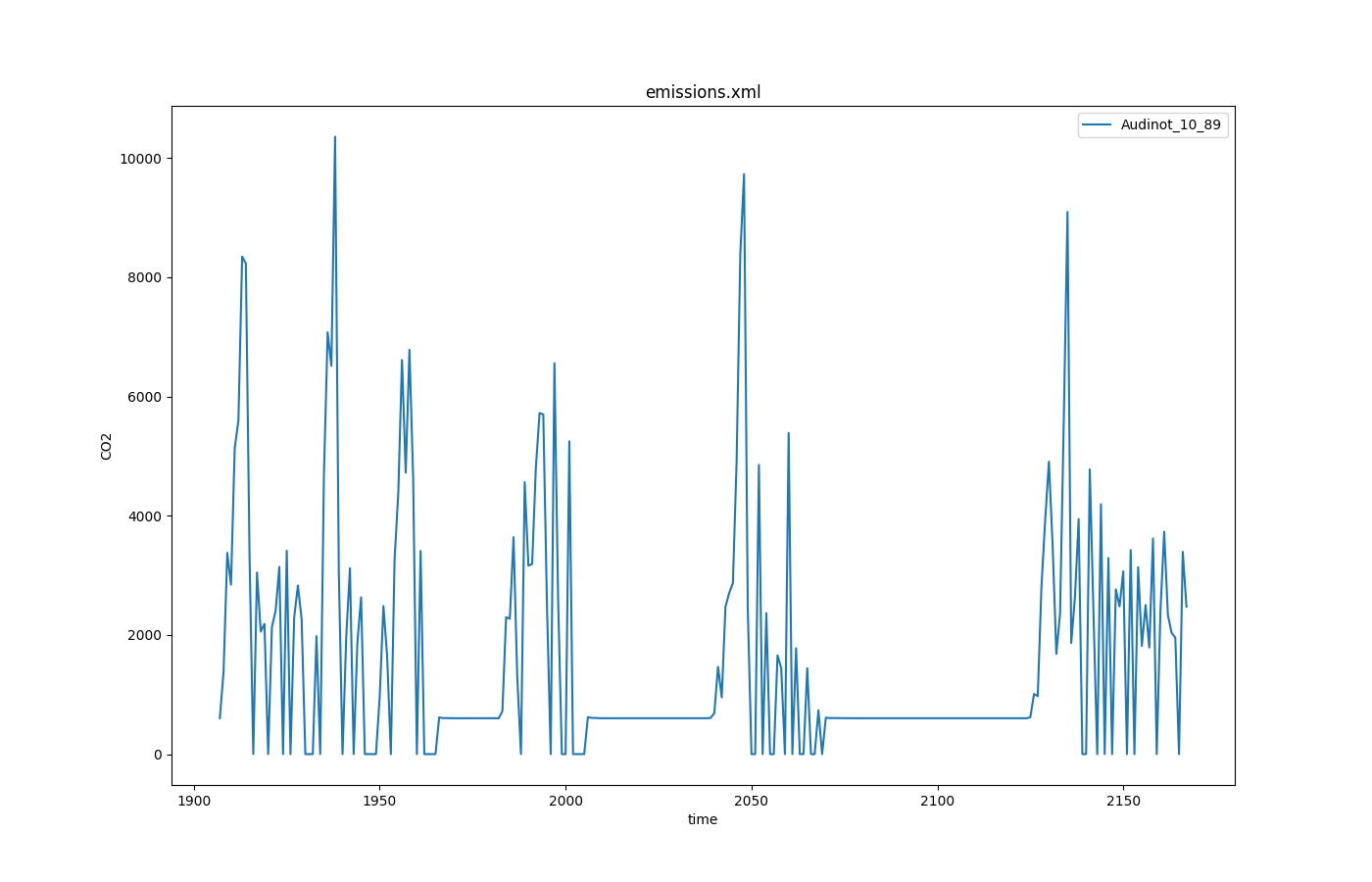EmissionOutput

The reason for this output option is the possibility of SUMO to calculate emission for every simulation timestep. The calculated values for every vehicle will be recorded and can be used for a more sophisticated view on special parts of the network. The values depend on the selected emission models for the individual vehicles.

## Instantiating within the Simulation#

To force SUMO to build a file that contains the emission output, extend your command line (or configuration) parameter by --emission-output <FILE>. <FILE> is the name of the file the output will be written to. Any other file with this name will be overwritten, the destination folder must exist.

By default, emission-output is enabled for all vehicles in the simulation with output in each simulation step. Using device assignment options or parameters (i.e. --device.emissions.probability 0.25) the set of vehicles which generate emission output can be reduced. The output period can be set by using option --device.emissions.period <TIME>. To delay output (i.e. until some warm-up time has passed), the option --device.emissions.begin <TIME> may be used.

## Generated Output#

Caution

Please note the the unit of fuel-related outputs changed with SUMO 1.14.0 from liters to milligram. For the old behavior use the option --emissions.volumetric-fuel.

The emission output is a xml-file containing the emission values for each time step for every vehicle. The produced XML File looks like this:

``````<emission-export>

<timestep time="<TIME_STEP>">

<vehicle id="<VEHICLE_ID>" eclass="<VEHICLE_EMISSION_CLASS>" CO2="<VEHICLE_CO2>" CO="<VEHICLE_CO>"
HC="<VEHICLE_HC>" NOx="<VEHICLE_NOX>" PMx="<VEHICLE_PMX>" fuel="<VEHICLE_FUEL>" electricity="<VEHICLE_ELECTRICITY>" noise="<VEHICLE_NOISE>" \
route="<VEHICLE_ROUTE>" type="<VEHICLE_TYPE>" waiting="<VEHICLE_WAITING>" lane="<VEHICLE_LANE>" \
pos="<VEHICLE_POS>" speed="<VEHICLE_SPEED>" angle="<VEHICLE_ANGLE>" x="<VEHICLE_POS_X>" y="<VEHICLE_POS_Y>"/>

... more vehicles ...

</timestep>

... the next timestep ...

</emission-export>
``````

The meanings of the written values are given in the following table.

Name Type Description
time_step (simulation) seconds The time step described by the values within this timestep-element
id id The id of the vehicle
eclass ID The emission class of the vehicle
CO2 mg/s The amount of CO2 emitted by the vehicle in the actual simulation step
CO mg/s The amount of CO emitted by the vehicle in the actual simulation step
HC mg/s The amount of HC emitted by the vehicle in the actual simulation step
NOx mg/s The amount of NOX emitted by the vehicle in the actual simulation step
PMx mg/s The amount of PMX emitted by the vehicle in the actual simulation step
fuel mg/s The amount of fuel used by the vehicle in the actual simulation step
electricity Wh/s The amount of electricity used by the vehicle in the actual simulation step
noise dB The noise emitted by the vehicle in the actual simulation step
route id The name of the route
type id The name of the vehicle type
waiting seconds The time the vehicle is waiting
lane id The name of the lane where the vehicle is moving
pos meters The vehicle position measured from the start of the current lane
speed m/s The speed of the vehicle
angle degree The angle of the vehicle
pos_x --- The absolut X coordinate of the vehicle. The value depends on the given geographic projection.
pos_y --- The absolut Y coordinate of the vehicle. The value depends on the given geographic projection.

Caution

When running with sub-second resolution, the emissions written during every simulation step are extrapolated to those that would be generated in 1 second. To avoid scaling (and thus simplify aggregation), set option --emission-output.step-scaled

## Visualization example#

The user-selected attributes can be plotted with use of plotXMLAttributes.py. The scenario acosta, one of the published sumo scenarios, is used as example (https://github.com/DLR-TS/sumo-scenarios/tree/main/bologna/acosta).

### Generating the output#

``````sumo -c run.sumocfg --emission-output emissions.xml
``````

### Example call for plotting#

``````python plotXMLAttributes.py -x time -y CO2 -s -o CO2_output.png emissions.xml -i id --filter-ids Audinot_10_89 --legend
``````

where -x is the attribute for the x axis; -y is the attribute for the y axis; -s is to show the plot; -o is the output file name; -i is the filtered attribute name; --filter-ids are the value(s) of the filtered attribute name; --legend is to show the value(s) of the selected attribute name.

The resultant plot is illustrated below.